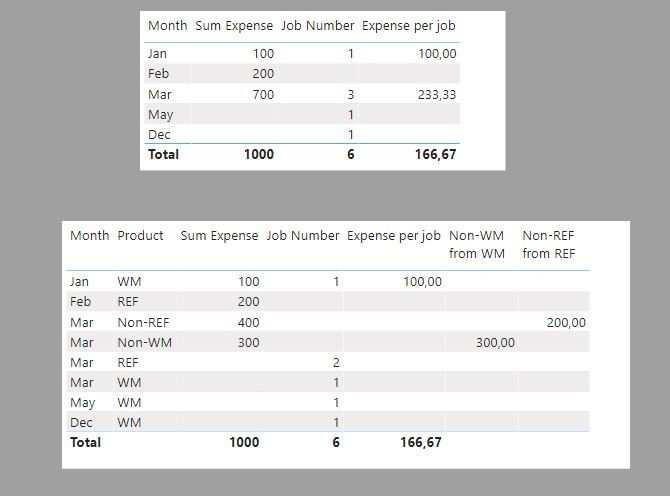cancel
Showing results for
Did you mean:Post Prodigy

## How to combine 2 tables into 1

Hi, I want to calculate the total expense per job by month based on the below 2 tables.

For example, the total expense for WM in January is \$100 and Job count in January is 2. Hence, \$100/2 = \$50.

For Product = Non-WM, I would like to take the total expense divide by Total Job Count for Product = WM.

Eg. In March, total expense for Non-WM is \$300 and total job count for WM = 1, so the expense per job = \$300/1 = \$300

Same goes to Product = Non-REF, I would take the total job count for product = REF as denominator.

Table A:

 Date Product Employee Expense 01/01/2021 WM ABC 100 02/25/2021 REF BCD 200 03/24/2021 Non-WM CDE 300 05/12/2021 Non-REF EFG 400

Table B:

 Date Job No. Product 01/05/2021 Job-123 WM 01/20/2021 Job-234 WM 02/12/2021 Job-345 WM 03/23/2021 Job-456 REF 05/03/2021 Job-457 REF
1 ACCEPTED SOLUTIONSuper User

I'm not sure if this is what you are after, and I have had to modify your tables to showcase the measures, but here we go...

With this model:and these measures:

``Sum Expense = SUM('Table A'[Expense])``
``Job Number = DISTINCTCOUNT('Table B'[Job No.])``
``````Expense per job =
VAR NumJobs = DISTINCTCOUNT('Table B'[Job No.])
RETURN
DIVIDE([Sum Expense], NumJobs)``````
``````Non-REF from REF =
VAR REF =
CALCULATE (
[Job Number],
'Dim Product'[Product] = "REF",
ALL ( 'Dim Product' )
)
RETURN
IF (
SELECTEDVALUE ( 'Dim Product'[Product] ) = "Non-REF",
DIVIDE ( [Sum Expense], REF )
)
``````
``````Non-WM from WM =
VAR WM =
CALCULATE ( [Job Number], 'Dim Product'[Product] = "WM", ALL ( 'Dim Product' ) )
RETURN
IF (
SELECTEDVALUE ( 'Dim Product'[Product] ) = "Non-WM",
DIVIDE ( [Sum Expense], WM )
)
``````

You get this...I've attached the sample PBIX file

In doing so, you are also helping me. Thank you!

Proud to be a Super User!

2 REPLIES 2Super User

I'm not sure if this is what you are after, and I have had to modify your tables to showcase the measures, but here we go...

With this model:and these measures:

``Sum Expense = SUM('Table A'[Expense])``
``Job Number = DISTINCTCOUNT('Table B'[Job No.])``
``````Expense per job =
VAR NumJobs = DISTINCTCOUNT('Table B'[Job No.])
RETURN
DIVIDE([Sum Expense], NumJobs)``````
``````Non-REF from REF =
VAR REF =
CALCULATE (
[Job Number],
'Dim Product'[Product] = "REF",
ALL ( 'Dim Product' )
)
RETURN
IF (
SELECTEDVALUE ( 'Dim Product'[Product] ) = "Non-REF",
DIVIDE ( [Sum Expense], REF )
)
``````
``````Non-WM from WM =
VAR WM =
CALCULATE ( [Job Number], 'Dim Product'[Product] = "WM", ALL ( 'Dim Product' ) )
RETURN
IF (
SELECTEDVALUE ( 'Dim Product'[Product] ) = "Non-WM",
DIVIDE ( [Sum Expense], WM )
)
``````

You get this...I've attached the sample PBIX file

In doing so, you are also helping me. Thank you!

Proud to be a Super User!Community Support

Hi @PBI_newuser ,

Why using count of WM for Non-WM and count of REF for Non-REF?

Best Regards,

Jay

Community Support Team _ Jay
If this post helps, then please consider Accept it as the solution
to help the other members find it.